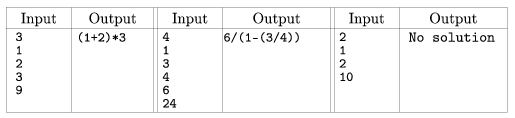Mathematical and Physical Journal
for High Schools
Issued by the MATFUND Foundation
 Already signed up? New to KöMaL?

#Problem I. 121. (January 2006)

I. 121. We are given some positive integers a1,...,ak and an integer s. Write a program to decide whether the number s can be represented using the numbers ai (each of them exactly once), the four basic operations and some parentheses.

If there is a solution, print one, otherwise display No solution''.

The program should read from the keyboard (standard input). The first line contains the value of k (k6), the next k lines contain the numbers ai, while the (k+2)th line contains the prescribed result s.

Examples:The source code of the program (i121.pas, i121.cpp, ...) should be submitted.

(10 pont)

Deadline expired on February 15, 2006.

### Statistics:

 11 students sent a solution. 10 points: Balambér Dávid, Czigler András, Györök Péter, Ozsvárt László, Szoldatics András. 6 points: 1 student. 4 points: 4 students. 0 point: 1 student.

Problems in Information Technology of KöMaL, January 2006